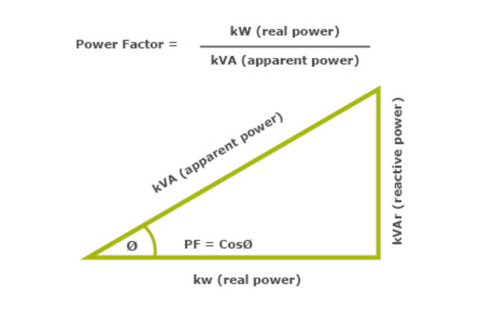Power Factor Correction

What is Power Factor?

Power quality is critical to the efficient operation of equipment. One contributing element to power quality is the power factor.

Power factor is the ratio of active (real) power kW divided by the total (apparent) power kVA.

In other words, power factor is a measure of how effectively incoming power is used in your electrical system and is defined as the ratio of Real to Apparent (total) power where:

• Real Power is the power that actually powers the equipment and performs useful productive work.
• Reactive Power is required by some equipment (eg. transformers, motors and relays) to produce a magnetic field for operation; however, it does not perform any real work.
• Apparent Power is the vector sum of Real and Reactive Power and corresponds to the total power required to produce the equivalent amount of real power for the load.Power Factor Correction may be required where a system has a power factor of less than 90% (or 0.9).

A poor power factor can contribute to equipment instability and failure, as well as significantly higher than necessary energy costs since it means that more current is required to perform the same amount of work.

By optimizing and improving the power factor, power quality is improved, reducing the load on the electricity distribution system.

PFC equipment achieves a decrease in the total amount of electrical demand by using a bank of capacitors to offset an inductive load (or reactors if the load is capacitive).See other examples below: